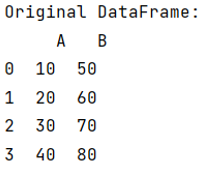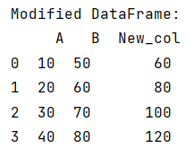# How to apply a function with multiple arguments to create a new Pandas column?

Given a Pandas DataFrame, we have to apply a function with multiple arguments. By Pranit Sharma Last updated : September 22, 2023

Pandas is a special tool which allows us to perform complex manipulations of data effectively and efficiently. Inside pandas, we mostly deal with a dataset in the form of DataFrame. DataFrames are 2-dimensional data structure in pandas. DataFrames consists of rows, columns and the data.

## Problem statement

Given a Pandas DataFrame, we have to apply a function with multiple arguments.

## Applying a function with multiple arguments to create a new Pandas column

We can insert a new column in a DataFrame whose values are defined from a function which takes multiple arguments. A simple comprehension function or lambda function can be used to continuously call the function with multiple arguments.

Note

To work with pandas, we need to import pandas package first, below is the syntax:

```import pandas as pd
```

Let us understand with the help of an example,

## Python program to apply a function with multiple arguments to create a new Pandas column

```# Importing pandas package
import pandas as pd

# Creating a dictionary
d = {"A": [10, 20, 30, 40], "B": [50, 60, 70, 80]}

# Creating a DataFrame
df = pd.DataFrame(d)

# Display the original DataFrame
print("Original DataFrame:\n", df, "\n")

# Defining a function
def fun(x, y):
return x + y

# Adding a new column into the DataFrame
df["New_col"] = df.apply(lambda x: fun(x["A"], x["B"]), axis=1)

# Display modified DataFrame
print("Modified DataFrame:\n", df)
```

### Output

The output of the above program is: